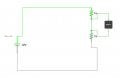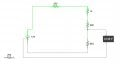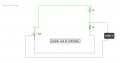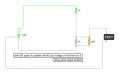# Design circuit that puts out 6v from 12v with no load

#### yescircuits

Joined Aug 29, 2015
20
Question on homework is:

Design and construct a circuit that puts out 6V (within 0.2V) from a 12-V power supply, with no load attached to the two output terminals. With a 500- ohm load attached, the output voltage drops to 3V (within 0.2V). Below is a diagram of a circuit that I built that should satisfy the first of this two part assignment but when I add a load of 500 ohms it does not drop to 3V. Any help would be greatly appreciated.#### ericgibbs

Joined Jan 29, 2010
18,283
hi y,
Where are you measuring the 'load' voltages on that circuit.?

Hint: assume the battery negative is the 0V reference for the load.

E

•yescircuits

#### atferrari

Joined Jan 6, 2004
4,730
Just in case, I built the same circuit in my bench and do not measure 6 V with my DMM connected as yours. Much less in fact.

•yescircuits

#### shteii01

Joined Feb 19, 2010
4,644
Last edited:
•yescircuits

#### yescircuits

Joined Aug 29, 2015
20
hi y,
Where are you measuring the 'load' voltages on that circuit.?

Hint: assume the battery negative is the 0V reference for the load.

E
I will first try making the correct v reading. I will send a new schematic

#### yescircuits

Joined Aug 29, 2015
20
Your volt meter is in the wrong place. The location where you have volt meter now, the volt meter should be showing 0 (zero) volts.
View attachment 90731

Photoshop?
Thanks will send new schematic soon

#### shteii01

Joined Feb 19, 2010
4,644
1.
Where is output voltage is taken?
Across 1k resistor?
Across 500 Ohm resistor?

2.
Do you know what Voltage Divider is?
Do you know how to calculate values of resistors needed to build Voltage Divider?

#### yescircuits

Joined Aug 29, 2015
20
In the above diagram the output voltage is taken across the 1kΩ resister. So part A of the assignment from 12V to 6V is correct?Would the above be an acceptable design for part two of the lab assignment? Or am I not able to split the resistors and re-position them?
I found my notes on voltage dividers...thanks for this help. Is there anything else that I need to know, for this assignment is due Monday night and I think I'm stressing over something simple.

#### JoeJester

Joined Apr 26, 2005
4,390
why not post a link to your assignment so everyone can see what you are suppose to do ....

that way the assistance provided would be tailored to your needs.

#### ericgibbs

Joined Jan 29, 2010
18,283
hi yes,
The question was, calculate the resistors to give 6V across the lower resistor, with No Load across the lower resistor.
Then it says add a 500 ohm Load across the lower resistor, ie: in parallel
What is the voltage now across the Load.? should be 3v.!!!

OK.
E

EDIT:

This is th question as I see it.
Part A, looks easy, but
Part B, with 500 ohm load will require some rethinking of Part A resistor values, in order to satisfy BOTH 6V and 3V , ie: without and with the 500 ohm Rload

Last edited:

#### yescircuits

Joined Aug 29, 2015
20
hi yes,
The question was, calculate the resistors to give 6V across the lower resistor, with No Load across the lower resistor.
Then it says add a 500 ohm Load across the lower resistor, ie: in parallel
What is the voltage now across the Load.? should be 3v.!!!

OK.
E

EDIT:

This is th question as I see it.
Part A, looks easy, but
Part B, with 500 ohm load will require some rethinking of Part A resistor values, in order to satisfy BOTH 6V and 3V , ie: without and with the 500 ohm Rload[/QUOTE

So it looks like I need a total of 5 resistors totaling 1.5k to solve, correct? Thanks for your help

#### yescircuits

Joined Aug 29, 2015
20
So it will take 5 resistors to solve, totaling 1.5k ohms? Thanks a lot for your help!

#### jjw

Joined Dec 24, 2013
818
So it will take 5 resistors to solve, totaling 1.5k ohms? Thanks a lot for your help!
No, it needs only 3 resistors.
From part A you get the relation of R1/R2.
In part B the load of 500 ohm is connected from the connection point of R1 and R2 to 0V ( parallel with R2 )
The voltage over 500 ohm is 3V and from there R1 R2 can be calculated.

•ericgibbs

#### yescircuits

Joined Aug 29, 2015
20
The below should wrap this assignment up. I am going to work on voltage dividers and this was a good jumpstart. Please let me know if the below two diagrams of the circuit fulfill the requirements, for I am going in to lab tonight at school to construct. Here is the assignment again:

Design and construct a circuit that puts out 6V (within 0.2V) from a 12-V power supply, with no load attached to the two output terminals. With a 500- ohm load attached, the output voltage drops to 3V (within 0.2V). Below is a diagram of a circuit that I built that should satisfy the first of this two part assignment but when I add a load of 500 ohms it does not drop to 3V. Any help would be greatly appreciated.#### jjw

Joined Dec 24, 2013
818
Why you say that the voltage does not drop to 3V in the second diagram? It seems to be 3V.
Is it enough that you have guessed the 1k resistor values or should you show how to calculate them?

#### yescircuits

Joined Aug 29, 2015
20
Why you say that the voltage does not drop to 3V in the second diagram? It seems to be 3V.
Is it enough that you have guessed the 1k resistor values or should you show how to calculate them?
My bad, that was a typo i ment to say it is 3v. Could you explain how you came up with the values please from the start. Thanks again!!!

#### yescircuits

Joined Aug 29, 2015
20
just want to make sure that I'm going about this forum correctly...am I? kind of new at that stuff

#### jjw

Joined Dec 24, 2013
818